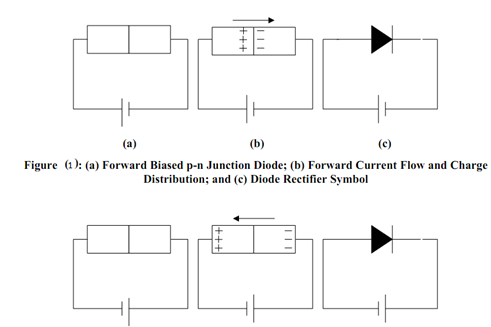## p-n Junction Diodes Assignment Help

Assignment Help: >> Diodes - p-n Junction Diodes

p-n Junction Diodes:

Diodes are semiconductor device, which force current to pass in only one direction. They are composed by connecting p-type and n-type semiconductors as illustrated in Figure 1(a) and Figure 2(a). While current flows from the p-type to the n-type semiconductors, the positive holes and the negative electrons are forced into close contact at the boundary. The electrons fill the holes across the boundary while the terminals supply new holes and electrons. Therefore, in the forward bias case of Figure 1(b) a continual current flows. In the reverse bias case, the charge carriers are pulled apart as illustrated in Figure 2(b). There is no longer an simple way for electrons to tunnel through the barrier, as there are no longer several empty holes waiting on the opposite side. The circuit-diagram representation of a diode is represented with an arrow representing the direction of current pass as depicted in Figure 1(c) and Figure 2(c).(a)                          (b)                                (c)

Figure: (a) Reverse Biased p-n Junction Diode; (b) Reverse Current Flow and Charge Distribution; and (c) Diode Rectifier Symbol